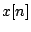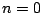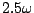Next: Classical waveforms Up: Fourier analysis and resynthesis Previous: Phase vocoder time bender   Contents   Index

# Exercises

1. A signalis 1 forandotherwise (an impulse). What is its (-point) Fourier transform as a function of?

2. Assuming further thatis an even number, what does the Fourier transform become ifis 1 atinstead of at?

3. For what integer values ofis the Fourier transform of the-point Hann window function nonzero?

4. In order to Fourier analyze a 100-Hertz periodic tone (at a sample rate of 44100 Hertz), using a Hann window, what value ofwould be needed to completely resolve all the partials of the tone (in the sense of having non-overlapping peaks in the spectrum)?

5. Suppose an N-point Fourier transform is done on a complex sinusoid of frequencywhereis the fundamental frequency. What percentage of the signal energy lands in the main lobe, channelsand? If the signal is Hann windowed, what percentage of the energy is now in the main lobe (which is then channels 1 through 4)?Next: Classical waveforms Up: Fourier analysis and resynthesis Previous: Phase vocoder time bender   Contents   Index
Miller Puckette 2006-12-30